Math Modeling : Multivariable Optimization

Example Questions

Example Question #1 : Multivariable Optimization

A company that makes computer monitors is unveiling two new products this spring, a 15 inch screen for $189 and a 19 inch screen for$269. The cost to manufacture the 15 inch screens is $85 per screen and the 19 inch screen is$99 per screen, plus \$200,000 for miscellaneous costs. The number of sells for each screen type effects the average selling price. It is estimated that each set average selling price drops by two cents for each additional screen sold. The average sells price for the 15 inch screen will decrease by 0.2 cents for each 19 inch screen sold, the price of the 19 inch screen decreases by 0.3 cents for each 15 inch screen sold. What are the partial differential equations used to find how many units of each type of screens should be manufactured to optimize profit?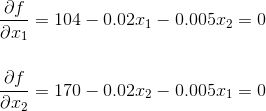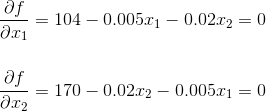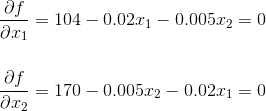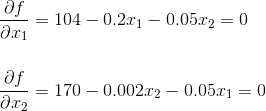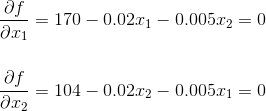Explanation:

First, identify all known information.

Variables: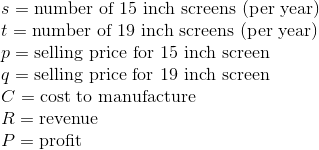Assumptions: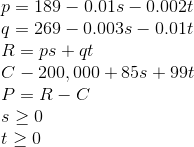Objective:

Maximize the profit.

Theorem: Ifis a function on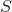which is a subset of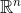andhas a minimum or maximum at an interior point inthen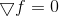Therefore, to find the maximum point in this function, simultaneously solve the differential equations in question and check the boundary points.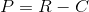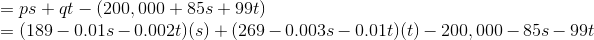Now switch variables/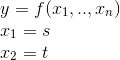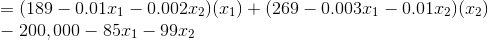Over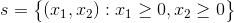From here take the partial differential equation of each variable. Since the questioned asked for the partial differential equations that would be used to optimize the profit we can stop here.